# Welcome to the Sets and Functions MCQs Page

Dive deep into the fascinating world of Sets and Functions with our comprehensive set of Multiple-Choice Questions (MCQs). This page is dedicated to exploring the fundamental concepts and intricacies of Sets and Functions, a crucial aspect of Discrete Mathematics. In this section, you will encounter a diverse range of MCQs that cover various aspects of Sets and Functions, from the basic principles to advanced topics. Each question is thoughtfully crafted to challenge your knowledge and deepen your understanding of this critical subcategory within Discrete Mathematics.

Check out the MCQs below to embark on an enriching journey through Sets and Functions. Test your knowledge, expand your horizons, and solidify your grasp on this vital area of Discrete Mathematics.

Note: Each MCQ comes with multiple answer choices. Select the most appropriate option and test your understanding of Sets and Functions. You can click on an option to test your knowledge before viewing the solution for a MCQ. Happy learning!

### Sets and Functions MCQs | Page 1 of 15

Q1.
The shaded area of figure is best described by?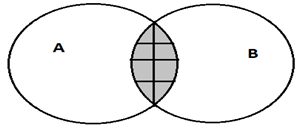Q2.
The shaded area of figure is best described by ?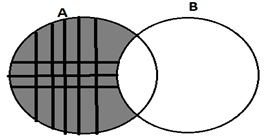Q3.
The shaded area of figure is best described by?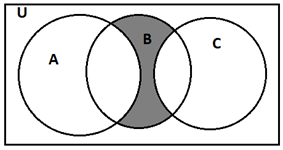Answer: (b).B – (A ∩ B) – (C ∩ B)
Q4.
In the given figure the if n(A)=20,n(U)=50,n(C)=10 and n(A∩B)=5 then n(B)=?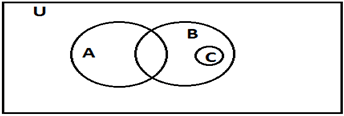Q5.
The shaded area of figure is best described by?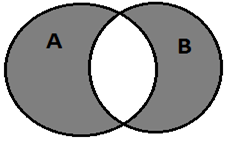Answer: (b).A U B – (A ∩ B)
Q6.
A function is defined by mapping f : A → B such that A contains m elements and B contains n elements and 1≤n≤m then number of onto functions are ________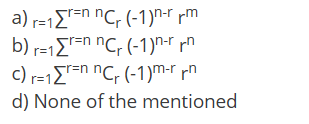a.

a

b.

b

c.

c

d.

d

Q7.
{x: x is an integer neither positive nor negative} is ________
Answer: (d).Non- empty and Finite set
Q8.
{x: x is a real number between 1 and 2} is an ________